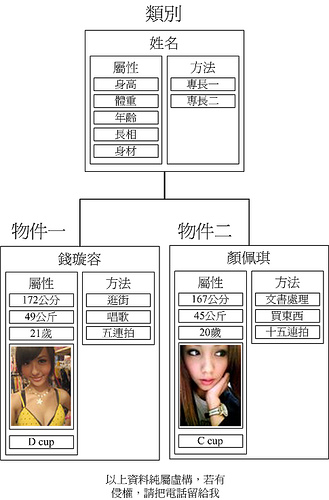#0

## 20點奉上!

，真的覺得自己進步好多阿，從一個物件類別搞不清楚的新手，到了現在變成微軟的技術顧問，

akitosun提到：akitosun提到：

summertw iT邦好手 1 級 ‧ 2015-01-30 08:50:24 檢舉
iT邦幫忙MVPwiselou提到：
『原雞』

Akito Sun iT邦新手 5 級 ‧ 2015-01-30 09:44:35 檢舉

Akito Sun iT邦新手 5 級 ‧ 2015-01-30 09:48:27 檢舉

Akito Sun iT邦新手 5 級 ‧ 2015-01-30 09:51:48 檢舉

Mitchell Moffit和Gregory Brown認為，先有雞或先有（雞）蛋的問題源自於「雞蛋」概念的歧義：如果「雞蛋」的定義是「孵出雞的蛋」，則演化上應是先由「原型雞」產下第一顆雞蛋，再由第一顆雞蛋孵出第一隻雞，故先有雞蛋。而如果「雞蛋」的定義是「由雞產下的蛋」，則演化上應是先由「原型雞」產下一顆基因變異的「原型雞蛋」，由該「原型雞蛋」孵出第一隻雞，再由第一隻雞產下第一顆雞蛋，故先有雞。
summertw iT邦好手 1 級 ‧ 2015-01-30 13:11:26 檢舉
akitosun提到：
「原型雞」==>「原型雞蛋」==>第一隻雞==>第一顆雞蛋，故先有雞。

Akito Sun iT邦新手 5 級 ‧ 2015-01-30 15:05:24 檢舉

### 5 個回答

7

``````&lt;pre class="c" name="code">
atk_a = str_a * 2 + int_a;
def_a = str_a + int_a * 2;

atk_b = str_b * 2 + int_b;
def_b = str_b + int_b * 2;

hp_a -= atk_b - def_a;
hp_b -= atk_a - def_b;
``````

``````&lt;pre class="c" name="code">
atk_a = str_array[a] * 2 + int_array[a];
def_a = str_array[a] + int_array[a] * 2;

atk_b = str_array[b] * 2 + int_array[b];
def_b = str_array[b] + int_array[b] * 2;

hp_array[a] -= atk_b - def_a;
hp_array[b] -= atk_a - def_b;
``````

``````&lt;pre class="c" name="code">
function calc_atk(str, int) {
return str * 2 + int;
}

function calc_def(str, int) {
return str + int * 2;
}

function calc_dmg(str_a, int_a, str_b, int_b) {
return calc_atk(str_a, str_b) - calc_def(str_b, int_b);
}

hp_array[a] -= calc_dmg(str_array[b], int_array[b], str_array[a], int_array[a]);
hp_array[b] -= calc_dmg(str_array[a], int_array[a], str_array[b], int_array[b]);
``````

``````&lt;pre class="c" name="code">
typedef struct character {
int str;
int intellect; // int是保留字, 所以改成 intellect
int hp;
} character;
// 然後在主程式裡就這樣寫:
character a, b;
``````

PHP 或其它語言可以用 array 或 hash:

``````&lt;pre class="c" name="code">
\$a = array(
"str" => 0,
"int" => 0,
"hp" => 0,
);
``````

``````&lt;pre class="c" name="code">
function calc_atk(char) {
return char.str * 2 + char.int;
}

function calc_def(char) {
return char.int * 2 + char.str;
}

function calc_dmg(attacker, defender) {
return calc_atk(attacker) - calc_def(defender);
}

a.hp -= calc_dmg(b, a);
b.hp -= calc_dmg(a, b);
``````

``````&lt;pre class="c" name="code">
class Character {
str;
int;
hp;

function calc_atk() {
return str * 2 + int;
}

function calc_def() {
return int * 2 + str;
}

function calc_dmg_to(defender) {
return calc_atk() - defender.calc_def();
}

function attack_to(defender) {
defender.hp -= calc_dmg_to(defender);
}
}

a.attack_to(b)
b.attack_to(a)
``````

``````&lt;pre class="c" name="code">
char_id = 0;
a = new Character(char_id++);
b = new Character(char_id++);
``````

``````&lt;pre class="c" name="code">
class Character {
static _id = 0;
char_id;
// 然後在建構式:
Character() {
char_id = _id++;
}
......

// 接著我們就不需要從外部給 id 了:
a = new Character(); // id = 0
b = new Character(); // id = 1
``````

``````&lt;pre class="c" name="code">
function get_harder(a, b) {
return a.calc_def() > b.calc_def() ? a : b;
}
``````

``````&lt;pre class="c" name="code">
class Character {
static function get_harder(char1, char2) {
return char1.calc_def() > char2.calc_def() ? char1 : char2;
}
......
// 然後在程式裡這樣使用:
use_char = Character.get_harder(a, b);
``````

pantc328 iT邦研究生 1 級 ‧ 2015-02-02 09:20:46 檢舉

weiclin提到：

pantc328 iT邦研究生 1 級 ‧ 2015-02-02 09:28:01 檢舉

weiclin提到：

int,boolean 也是物件,宣告時會自己產生物件在堆疊的位置,參照和預設值
Class 轉物件需要實例化,會在Hash配置一塊記億體空間,然後物件指標指到Hash位置

6

fillano iT邦超人 1 級 ‧ 2015-01-29 16:27:01 檢舉5

OOP，市面上有很多書，很多老師在講（包含學校也上過課）

http://www.allenkuo.com/edm/OOP201404.html

http://www.dotblogs.com.tw/mis2000lab/archive/2014/06/16/mvc\_or\_web\_form\_20140616.aspx

0

0

public class Car
{
public int speed{};
public float gas{};
}

public void Main(....)
{
Car MyCar=new Car();
Car girlfirendCar=new Car();
}

................